# Closest natural number

Find the closest natural number to 4.456 to 44.56 and 445.6.

a =  4
b =  45
c =  446

### Step-by-step explanation:

$a=\left[4.456\right]=4$
$b=\left[44.56\right]=45$
$c=\left[445.6\right]=446$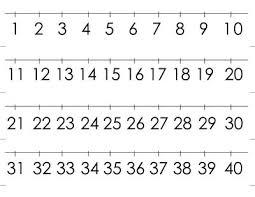Did you find an error or inaccuracy? Feel free to write us. Thank you!Tips to related online calculators
Do you want to round the number?

## Related math problems and questions:

• Four multiplesFour multiples of 6 he writes the following 12  24  56  72, which is correct?
• Divisible by fourAre 240 divisible by 4?
• RemaindersIt is given a set of numbers { 170; 244; 299; 333; 351; 391; 423; 644 }. Divide this numbers by number 66 and determine set of remainders. As result write sum of this remainders.
• How manyHow many integers are greater than 547/3 and less than 931/4?
• Gamma angleFind the magnitude of the gamma angle in triangle ABC if: α = 38° 56 ’and β = 47° 54’.
• Cash back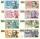After a trip 24 students have left more than 650 but less than 690 CZK (Kc). How much each student receives return when the amount is divisible by the number of pupils?
• Sum of four numbers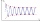The sum of four consecutive natural numbers is 114. Find them.
• Speed of Slovakian trainsRudolf decided to take the train from the station 'Ostratice' to 'Horné Ozorovce'. In the train timetables found train Os 5409 : km 0 Chynorany 15:17 5 Ostratice 15:23 15:23 8 Rybany 15:27 15:27 10 Dolné Naštice 15:31 15:31 14 Bánovce nad Bebravou 15:35 1
• FractionFind for what x fraction (-4x -6)/(x) equals:
• Rectangle 39Find the perimeter and area of the rectangular with vertices (-1, 4), (0,4), (0, -1), and (-4, 4)
• Find unknown numberWhat is the number between 50 and 55 that is divisible by 2,3,6,9?
• Area 4gonCalculate the area of 4-gon, two, and the two sides are equal and parallel with lengths 11, 5, 11, and 5. Inner angles are 45°, 135°,45°, 135°.
• Rounding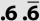Double round number 727, first to tens, then to hundreds. (double rounding)
• Unknown number4/5 of a number is 276. what is 2/3 of the same number?
• I think numberI think number.When I add 841 to it and subtract 157, I get a number that is 22 greater than 996. What number I thinking?
• By sixFrom the digits 1,2,3,4 we create the long integer number 123412341234. .. .. , which will have 962 digits. Is this number divisible by 6?
• Brian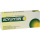Brian divides 80 by 7. Between what two whole numbers is his answer?